### Input data:

Number of nodes in the circuit

### D i e s e l s

Number of diesels (to 2)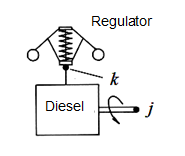The diesel structural parameters

Physical, geometrical and design parameters of the diesel

No

Nodes numbers

Inertia
moment
of
rotating
details

kg.m2
Torque
increment
at maximal
fuel
supply

N.m
Coeff.of
viscous
friction
in
diesel
regulator

N.s/m
Coefficients of diesel regulator setting

Gear
ratio
of
regulator
drive

-
Rigidity
of
regulator
spring

N/m
Force of
regulator
spring
prelim.
compres-
sion

N
Maximal
stroke
of
regulator
muff

mm

i

j

k
ke

N
b

N.s2
k

N.s2/m
The    d i e s e l    c h a r a c t e r i s t i c    Мe ( ωj ).
ωj - an angular velocity of the diesel shaft (in node j ), rad/s;    Мe - the diesel shaft torque, N.m .

ω1 ω2 ω3 ω4 ω5 ω6 ω7 ω8 ω9 ω10
M1 M2 M3 M4 M5 M6 M7 M8 M9 M10

The diesel structural parameters

Physical, geometrical and design parameters of the diesel

No

Nodes numbers

Inertia
moment
of
rotating
details

kg.m2
Torque
increment
at maximal
fuel
supply

N.m
Coeff.of
viscous
friction
in
diesel
regulator

N.s/m
Coefficients of diesel regulator setting

Gear
ratio
of
regulator
drive

-
Rigidity
of
regulator
spring

N/m
Force of
regulator
spring
prelim.
compres-
sion

N
Maximal
stroke
of
regulator
muff

mm

i

j

k
ke

N
b

N.s2
k

N.s2/m
The    d i e s e l    c h a r a c t e r i s t i c    Мe ( ωj ).
ωj - an angular velocity of the diesel shaft (in node j ), rad/s;    Мe - the diesel shaft torque, N.m .

ω1 ω2 ω3 ω4 ω5 ω6 ω7 ω8 ω9 ω10
M1 M2 M3 M4 M5 M6 M7 M8 M9 M10

### G e a r b o x e s

Number of gearboxes (to 10)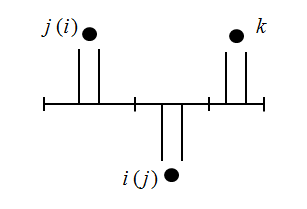The gearbox structural parameters

Physical, geometrical and design parameters of the gearbox

No
Nodes numbers

Inertia
moment
in node i

kg.m2
Inertia
moment
in node j

kg.m2
Inertia
moment
in node k

kg.m2
Ratio
of
branch i-j

-
Ratio
of
branch i-k

-
Efficiency
of
branch i-j

-
Efficiency
of
branch i-j

-
Nominal
torque of
branch i-j

N.m
Nominal
torque of
branch i-j

N.m
i j k

### E l a s t i c   s h a f t s

Number of elastic shafts (to 10)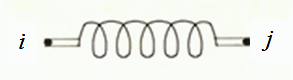The elastic shaft structural parameters

Physical, geometrical and design parameters of the elastic shaft

No
Nodes numbers

Torsional
stiffness

N.m
Coefficient
of viscous
friction

N.m.s
i j k

### F r i c t i o n a l    c l u t c h e s

Number of frictional clutches (to 10)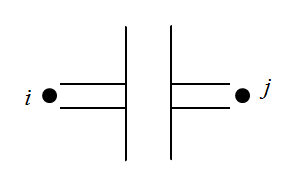The frictional clutch structural parameters

Physical, geometrical and design parameters of the frictional clutch

No
Nodes numbers

Inertia
moment
disk

kg.m2
Inertia
moment
of driven
disk

kg.m2
Const-
ructive
constant

m3
i j k
F r i c t i o n a l    c l u t c h    c h a r a c t e r i s t i c    μ ( ∆ω ).
∆ω - difference between angular velocities of the friction disks, rad/s;    μ - coefficient of friction.

∆ω1 ∆ω2 ∆ω3 ∆ω4 ∆ω5 ∆ω6 ∆ω7 ∆ω8 ∆ω9 ∆ω10
μ1 μ2 μ3 μ4 μ5 μ6 μ7 μ8 μ9 μ10

The frictional clutch structural parameters

Physical, geometrical and design parameters of the frictional clutch

No
Nodes numbers

Inertia
moment
disk

kg.m2
Inertia
moment
of driven
disk

kg.m2
Const-
ructive
constant

m3
i j k
F r i c t i o n a l    c l u t c h    c h a r a c t e r i s t i c    μ ( ∆ω ).
∆ω - difference between angular velocities of the friction disks, rad/s;    μ - coefficient of friction.

∆ω1 ∆ω2 ∆ω3 ∆ω4 ∆ω5 ∆ω6 ∆ω7 ∆ω8 ∆ω9 ∆ω10
μ1 μ2 μ3 μ4 μ5 μ6 μ7 μ8 μ9 μ10

The frictional clutch structural parameters

Physical, geometrical and design parameters of the frictional clutch

No
Nodes numbers

Inertia
moment
disk

kg.m2
Inertia
moment
of driven
disk

kg.m2
Const-
ructive
constant

m3
i j k
F r i c t i o n a l    c l u t c h    c h a r a c t e r i s t i c    μ ( ∆ω ).
∆ω - difference between angular velocities of the friction disks, rad/s;    μ - coefficient of friction.

∆ω1 ∆ω2 ∆ω3 ∆ω4 ∆ω5 ∆ω6 ∆ω7 ∆ω8 ∆ω9 ∆ω10
μ1 μ2 μ3 μ4 μ5 μ6 μ7 μ8 μ9 μ10

The frictional clutch structural parameters

Physical, geometrical and design parameters of the frictional clutch

No
Nodes numbers

Inertia
moment
disk

kg.m2
Inertia
moment
of driven
disk

kg.m2
Const-
ructive
constant

m3
i j k
F r i c t i o n a l    c l u t c h    c h a r a c t e r i s t i c    μ ( ∆ω ).
∆ω - difference between angular velocities of the friction disks, rad/s;    μ - coefficient of friction.

∆ω1 ∆ω2 ∆ω3 ∆ω4 ∆ω5 ∆ω6 ∆ω7 ∆ω8 ∆ω9 ∆ω10
μ1 μ2 μ3 μ4 μ5 μ6 μ7 μ8 μ9 μ10

The frictional clutch structural parameters

Physical, geometrical and design parameters of the frictional clutch

No
Nodes numbers

Inertia
moment
disk

kg.m2
Inertia
moment
of driven
disk

kg.m2
Const-
ructive
constant

m3
i j k
F r i c t i o n a l    c l u t c h    c h a r a c t e r i s t i c    μ ( ∆ω ).
∆ω - difference between angular velocities of the friction disks, rad/s;    μ - coefficient of friction.

∆ω1 ∆ω2 ∆ω3 ∆ω4 ∆ω5 ∆ω6 ∆ω7 ∆ω8 ∆ω9 ∆ω10
μ1 μ2 μ3 μ4 μ5 μ6 μ7 μ8 μ9 μ10

The frictional clutch structural parameters

Physical, geometrical and design parameters of the frictional clutch

No
Nodes numbers

Inertia
moment
disk

kg.m2
Inertia
moment
of driven
disk

kg.m2
Const-
ructive
constant

m3
i j k
F r i c t i o n a l    c l u t c h    c h a r a c t e r i s t i c    μ ( ∆ω ).
∆ω - difference between angular velocities of the friction disks, rad/s;    μ - coefficient of friction.

∆ω1 ∆ω2 ∆ω3 ∆ω4 ∆ω5 ∆ω6 ∆ω7 ∆ω8 ∆ω9 ∆ω10
μ1 μ2 μ3 μ4 μ5 μ6 μ7 μ8 μ9 μ10

The frictional clutch structural parameters

Physical, geometrical and design parameters of the frictional clutch

No
Nodes numbers

Inertia
moment
disk

kg.m2
Inertia
moment
of driven
disk

kg.m2
Const-
ructive
constant

m3
i j k
F r i c t i o n a l    c l u t c h    c h a r a c t e r i s t i c    μ ( ∆ω ).
∆ω - difference between angular velocities of the friction disks, rad/s;    μ - coefficient of friction.

∆ω1 ∆ω2 ∆ω3 ∆ω4 ∆ω5 ∆ω6 ∆ω7 ∆ω8 ∆ω9 ∆ω10
μ1 μ2 μ3 μ4 μ5 μ6 μ7 μ8 μ9 μ10

The frictional clutch structural parameters

Physical, geometrical and design parameters of the frictional clutch

No
Nodes numbers

Inertia
moment
disk

kg.m2
Inertia
moment
of driven
disk

kg.m2
Const-
ructive
constant

m3
i j k
F r i c t i o n a l    c l u t c h    c h a r a c t e r i s t i c    μ ( ∆ω ).
∆ω - difference between angular velocities of the friction disks, rad/s;    μ - coefficient of friction.

∆ω1 ∆ω2 ∆ω3 ∆ω4 ∆ω5 ∆ω6 ∆ω7 ∆ω8 ∆ω9 ∆ω10
μ1 μ2 μ3 μ4 μ5 μ6 μ7 μ8 μ9 μ10

The frictional clutch structural parameters

Physical, geometrical and design parameters of the frictional clutch

No
Nodes numbers

Inertia
moment
disk

kg.m2
Inertia
moment
of driven
disk

kg.m2
Const-
ructive
constant

m3
i j k
F r i c t i o n a l    c l u t c h    c h a r a c t e r i s t i c    μ ( ∆ω ).
∆ω - difference between angular velocities of the friction disks, rad/s;    μ - coefficient of friction.

∆ω1 ∆ω2 ∆ω3 ∆ω4 ∆ω5 ∆ω6 ∆ω7 ∆ω8 ∆ω9 ∆ω10
μ1 μ2 μ3 μ4 μ5 μ6 μ7 μ8 μ9 μ10

The frictional clutch structural parameters

Physical, geometrical and design parameters of the frictional clutch

No
Nodes numbers

Inertia
moment
disk

kg.m2
Inertia
moment
of driven
disk

kg.m2
Const-
ructive
constant

m3
i j k
F r i c t i o n a l    c l u t c h    c h a r a c t e r i s t i c    μ ( ∆ω ).
∆ω - difference between angular velocities of the friction disks, rad/s;    μ - coefficient of friction.

∆ω1 ∆ω2 ∆ω3 ∆ω4 ∆ω5 ∆ω6 ∆ω7 ∆ω8 ∆ω9 ∆ω10
μ1 μ2 μ3 μ4 μ5 μ6 μ7 μ8 μ9 μ10

### H y d r o    d y n a m i c a l    c l u t c h e s

Number of hydro dynamical clutches (to 3)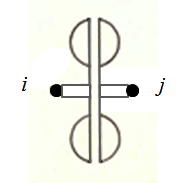The hydro dynamical clutch structural parameters

Physical, geometrical and design parameters of hydro dynamical clutch

No
Nodes numbers

Inertia
moment
of pump
impeller

kg.m2
Inertia
moment
of turbine
impeller

kg.m2
Diameter
of working
wheels

m
i j k
H y d r o    d y n a m i c a l    c l u t c h    c h a r a c t e r i s t i c    104.ρλ ( ic ).
ic - gear ratio;    104.ρλ - coefficient of moment.

iм 1 iм 2 iм 3 iм 4 iм 5 iм 6 iм 7 iм 8 iм 9 iм 10
104.ρλ1 104.ρλ2 104.ρλ3 104.ρλ4 104.ρλ5 104.ρλ6 104.ρλ7 104.ρλ8 104.ρλ9 104.ρλ10

The hydro dynamical clutch structural parameters

Physical, geometrical and design parameters of hydro dynamical clutch

No
Nodes numbers

Inertia
moment
of pump
impeller

kg.m2
Inertia
moment
of turbine
impeller

kg.m2
Diameter
of working
wheels

m
i j k
H y d r o    d y n a m i c a l    c l u t c h    c h a r a c t e r i s t i c    104.ρλ ( ic ).
ic - gear ratio;    104.ρλ - coefficient of moment.

iм 1 iм 2 iм 3 iм 4 iм 5 iм 6 iм 7 iм 8 iм 9 iм 10
104.ρλ1 104.ρλ2 104.ρλ3 104.ρλ4 104.ρλ5 104.ρλ6 104.ρλ7 104.ρλ8 104.ρλ9 104.ρλ10

The hydro dynamical clutch structural parameters

Physical, geometrical and design parameters of hydro dynamical clutch

No
Nodes numbers

Inertia
moment
of pump
impeller

kg.m2
Inertia
moment
of turbine
impeller

kg.m2
Diameter
of working
wheels

m
i j k
H y d r o    d y n a m i c a l    c l u t c h    c h a r a c t e r i s t i c    104.ρλ ( ic ).
ic - gear ratio;    104.ρλ - coefficient of moment.

iм 1 iм 2 iм 3 iм 4 iм 5 iм 6 iм 7 iм 8 iм 9 iм 10
104.ρλ1 104.ρλ2 104.ρλ3 104.ρλ4 104.ρλ5 104.ρλ6 104.ρλ7 104.ρλ8 104.ρλ9 104.ρλ10

### H y d r a u l i c    t o r q u e    c o n v e r t e r s    (HTC)

Number of torque converters (to 3)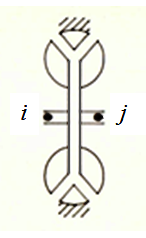ПРИМЕЧАНИЯ: 1. Field *) is filled, if the HTC impellers are blocked by the fructional clutch. Otherwise, put 0.
2. If HTC contains overtaking clutch, in field **) is 1, otherwise 0.

The hydrulic torque converter structural parameters

Physical, geometrical and design parameters of hydrulic torque converter

No
Nodes numbers

Inertia
moment
of pump
impeller

kg.m2
Inertia
moment
of turbine
impeller

kg.m2
Diameter
of working
wheels

m
Constructive
constant
of fructional
clutch *)

м3
Sign of
overtaking
clutch**)
blocking
-
i j k
H y d r a u l i c    t o r q u e    c o n v e r t e r    c h a r a c t e r i s t i c    104.ρλ1,2 ( it )
it - gear ratio;    104.ρλ1,2 - coefficients of moments of pump and turbine impellers.

P u m p    i m p e l l e r
iт 1 iт 2 iт 3 iт 4 iт 5 iт 6 iт 7 iт 8 iт 9 iт 10
104.ρλ1,1 104.ρλ1,2 104.ρλ1,3 104.ρλ1,4 104.ρλ1,5 104.ρλ1,6 104.ρλ1,7 104.ρλ1,8 104.ρλ1,9 104.ρλ1,10
T u r b i n e    i m p e l l e r
iт 1 iт 2 iт 3 iт 4 iт 5 iт 6 iт 7 iт 8 iт 9 iт 10
104.ρλ2,1 104.ρλ2,2 104.ρλ2,3 104.ρλ2,4 104.ρλ2,5 104.ρλ2,6 104.ρλ2,7 104.ρλ2,8 104.ρλ2,9 104.ρλ2,10
B l o c k i n g    f r i c t i o n a l    c l u t c h    c h a r a c t e r i s t i c    μ ( ∆ω ).
∆ω - difference between angular velocities of the friction disks, rad/s;    μ - coefficient of friction.

∆ω1 ∆ω2 ∆ω3 ∆ω4 ∆ω5 ∆ω6 ∆ω7 ∆ω8 ∆ω9 ∆ω10
μ1 μ2 μ3 μ4 μ5 μ6 μ7 μ8 μ9 μ10

The hydrulic torque converter structural parameters

Physical, geometrical and design parameters of hydrulic torque converter

No
Nodes numbers

Inertia
moment
of pump
impeller

kg.m2
Inertia
moment
of turbine
impeller

kg.m2
Diameter
of working
wheels

m
Constructive
constant
of fructional
clutch *)

м3
Sign of
overtaking
clutch**)
blocking
-
i j k
H y d r a u l i c    t o r q u e    c o n v e r t e r    c h a r a c t e r i s t i c    104.ρλ1,2 ( it )
it - gear ratio;    104.ρλ1,2 - coefficients of moments of pump and turbine impellers.

P u m p    i m p e l l e r
iт 1 iт 2 iт 3 iт 4 iт 5 iт 6 iт 7 iт 8 iт 9 iт 10
104.ρλ1,1 104.ρλ1,2 104.ρλ1,3 104.ρλ1,4 104.ρλ1,5 104.ρλ1,6 104.ρλ1,7 104.ρλ1,8 104.ρλ1,9 104.ρλ1,10
T u r b i n e    i m p e l l e r
iт 1 iт 2 iт 3 iт 4 iт 5 iт 6 iт 7 iт 8 iт 9 iт 10
104.ρλ2,1 104.ρλ2,2 104.ρλ2,3 104.ρλ2,4 104.ρλ2,5 104.ρλ2,6 104.ρλ2,7 104.ρλ2,8 104.ρλ2,9 104.ρλ2,10
B l o c k i n g    f r i c t i o n a l    c l u t c h    c h a r a c t e r i s t i c    μ ( ∆ω ).
∆ω - difference between angular velocities of the friction disks, rad/s;    μ - coefficient of friction.

∆ω1 ∆ω2 ∆ω3 ∆ω4 ∆ω5 ∆ω6 ∆ω7 ∆ω8 ∆ω9 ∆ω10
μ1 μ2 μ3 μ4 μ5 μ6 μ7 μ8 μ9 μ10

The hydrulic torque converter structural parameters

Physical, geometrical and design parameters of hydrulic torque converter

No
Nodes numbers

Inertia
moment
of pump
impeller

kg.m2
Inertia
moment
of turbine
impeller

kg.m2
Diameter
of working
wheels

m
Constructive
constant
of fructional
clutch *)

м3
Sign of
overtaking
clutch**)
blocking
-
i j k
H y d r a u l i c    t o r q u e    c o n v e r t e r    c h a r a c t e r i s t i c    104.ρλ1,2 ( it )
it - gear ratio;    104.ρλ1,2 - coefficients of moments of pump and turbine impellers.

P u m p    i m p e l l e r
iт 1 iт 2 iт 3 iт 4 iт 5 iт 6 iт 7 iт 8 iт 9 iт 10
104.ρλ1,1 104.ρλ1,2 104.ρλ1,3 104.ρλ1,4 104.ρλ1,5 104.ρλ1,6 104.ρλ1,7 104.ρλ1,8 104.ρλ1,9 104.ρλ1,10
T u r b i n e    i m p e l l e r
iт 1 iт 2 iт 3 iт 4 iт 5 iт 6 iт 7 iт 8 iт 9 iт 10
104.ρλ2,1 104.ρλ2,2 104.ρλ2,3 104.ρλ2,4 104.ρλ2,5 104.ρλ2,6 104.ρλ2,7 104.ρλ2,8 104.ρλ2,9 104.ρλ2,10
B l o c k i n g    f r i c t i o n a l    c l u t c h    c h a r a c t e r i s t i c    μ ( ∆ω ).
∆ω - difference between angular velocities of the friction disks, rad/s;    μ - coefficient of friction.

∆ω1 ∆ω2 ∆ω3 ∆ω4 ∆ω5 ∆ω6 ∆ω7 ∆ω8 ∆ω9 ∆ω10
μ1 μ2 μ3 μ4 μ5 μ6 μ7 μ8 μ9 μ10

### F l y w h e e l s

Number of flywheels (to 10)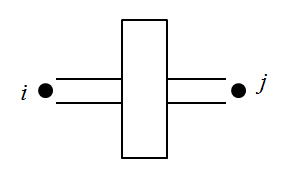The flywheel
structural
parameters

Physical
parameter
of
flywheel

No
Nodes numbers

Inertia
moment

kg.m2
i j k

### W h e e l    m o v e r

Number of wheels (axles) (to 8)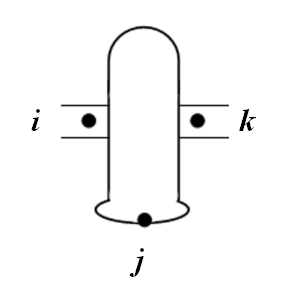NOTE: This element is either a wheel with independent drive, or driving axle.

The wheel structural parameters

Physical, geometric and structural
parameters of wheel

No
Nodes numbers

Inertia
moment

kg.m2
Free
of wheel

m
Vertical
on axle
(wheel)
N
stiffness
of tire

N/m
Circum-
ferential
stiffness
of tire
N/m
i j k
W h e e l    s l i p p i n g    c u r v e    R ( δ ).
δ - relative slipping, dimensionless;   R - circumferential force on wheel, N.

δ1 δ2 δ3 δ4 δ5 δ6 δ7 δ8 δ9 δ10
R1 R2 R3 R4 R5 R6 R7 R8 R9 R10

The wheel structural parameters

Physical, geometric and structural
parameters of wheel

No
Nodes numbers

Inertia
moment

kg.m2
Free
of wheel

m
Vertical
on axle
(wheel)
N
stiffness
of tire

N/m
Circum-
ferential
stiffness
of tire
N/m
i j k
W h e e l    s l i p p i n g    c u r v e    R ( δ ).
δ - relative slipping, dimensionless;   R - circumferential force on wheel, N.

δ1 δ2 δ3 δ4 δ5 δ6 δ7 δ8 δ9 δ10
R1 R2 R3 R4 R5 R6 R7 R8 R9 R10

The wheel structural parameters

Physical, geometric and structural
parameters of wheel

No
Nodes numbers

Inertia
moment

kg.m2
Free
of wheel

m
Vertical
on axle
(wheel)
N
stiffness
of tire

N/m
Circum-
ferential
stiffness
of tire
N/m
i j k
W h e e l    s l i p p i n g    c u r v e    R ( δ ).
δ - relative slipping, dimensionless;   R - circumferential force on wheel, N.

δ1 δ2 δ3 δ4 δ5 δ6 δ7 δ8 δ9 δ10
R1 R2 R3 R4 R5 R6 R7 R8 R9 R10

The wheel structural parameters

Physical, geometric and structural
parameters of wheel

No
Nodes numbers

Inertia
moment

kg.m2
Free
of wheel

m
Vertical
on axle
(wheel)
N
stiffness
of tire

N/m
Circum-
ferential
stiffness
of tire
N/m
i j k
W h e e l    s l i p p i n g    c u r v e    R ( δ ).
δ - relative slipping, dimensionless;   R - circumferential force on wheel, N.

δ1 δ2 δ3 δ4 δ5 δ6 δ7 δ8 δ9 δ10
R1 R2 R3 R4 R5 R6 R7 R8 R9 R10

The wheel structural parameters

Physical, geometric and structural
parameters of wheel

No
Nodes numbers

Inertia
moment

kg.m2
Free
of wheel

m
Vertical
on axle
(wheel)
N
stiffness
of tire

N/m
Circum-
ferential
stiffness
of tire
N/m
i j k
W h e e l    s l i p p i n g    c u r v e    R ( δ ).
δ - relative slipping, dimensionless;   R - circumferential force on wheel, N.

δ1 δ2 δ3 δ4 δ5 δ6 δ7 δ8 δ9 δ10
R1 R2 R3 R4 R5 R6 R7 R8 R9 R10

The wheel structural parameters

Physical, geometric and structural
parameters of wheel

No
Nodes numbers

Inertia
moment

kg.m2
Free
of wheel

m
Vertical
on axle
(wheel)
N
stiffness
of tire

N/m
Circum-
ferential
stiffness
of tire
N/m
i j k
W h e e l    s l i p p i n g    c u r v e    R ( δ ).
δ - relative slipping, dimensionless;   R - circumferential force on wheel, N.

δ1 δ2 δ3 δ4 δ5 δ6 δ7 δ8 δ9 δ10
R1 R2 R3 R4 R5 R6 R7 R8 R9 R10

The wheel structural parameters

Physical, geometric and structural
parameters of wheel

No
Nodes numbers

Inertia
moment

kg.m2
Free
of wheel

m
Vertical
on axle
(wheel)
N
stiffness
of tire

N/m
Circum-
ferential
stiffness
of tire
N/m
i j k
W h e e l    s l i p p i n g    c u r v e    R ( δ ).
δ - relative slipping, dimensionless;   R - circumferential force on wheel, N.

δ1 δ2 δ3 δ4 δ5 δ6 δ7 δ8 δ9 δ10
R1 R2 R3 R4 R5 R6 R7 R8 R9 R10

The wheel structural parameters

Physical, geometric and structural
parameters of wheel

No
Nodes numbers

Inertia
moment

kg.m2
Free
of wheel

m
Vertical
on axle
(wheel)
N
stiffness
of tire

N/m
Circum-
ferential
stiffness
of tire
N/m
i j k
W h e e l    s l i p p i n g    c u r v e    R ( δ ).
δ - relative slipping, dimensionless;   R - circumferential force on wheel, N.

δ1 δ2 δ3 δ4 δ5 δ6 δ7 δ8 δ9 δ10
R1 R2 R3 R4 R5 R6 R7 R8 R9 R10

### D i f f e r e n t i a l s

Number of differentials (to 8)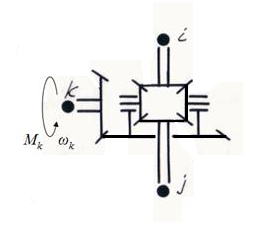The differential structural parameters

Physical, geometric and structural
parameters of differential

No
Nodes numbers

Inertia
moment of
semiaxis
in node i
kg.m2
Inertia
moment of
semiaxis
in node i j
kg.m2
Inertia
moment of
pinion
in node i k
kg.m2
Inertia
moment of
carrier

kg.m2
Gear
ratio

-
Gear
ratio of
diffe-
rential
-
i j k

Vehicle mass, kg

### E x t e r n a l    p e r t u r b a t i o n s    and    c o n t r o l    s i g n a l s

Number of perturbations (to 10)

 Type of perturbation:      1 - control pressure of N-th friction clutch as function of time, MPa;      2 - control pressure of friction clutch of N-th hydraulic torque converter as function of time, MPa;      3 - dynamic deflection of N-th wheel tire due to impact of road profile as function of traversed path, m;      4 - braking torque in node N as function of time, N.m;      5 - resistance to machine movement in node N, taking into account the longitudinal gradient of the road as function of traversed path, N;      6 - external moment in node N as function of time, N.m;      7 - control of gas pedal of N-th diesel as function of time ψ(t), 0 ≤ ψ(t) ≤ 1.      N - element number, if type of perturbation 1, 2, 3, or 7;      N - node number, if type of perturbation 4, 5 or 6.

#### Perturbation No 1

Type of perturbation   Element number or node number where the given perturbation acts

F u n c t i o n    of    e x t e r n a l    p e r t u r b a t i o n    y ( x ).
xi - i-st value of argument;    yi - i-st value of function.

x1 x2 x3 x4 x5 x6 x7 x8 x9 x10
y1 y2 y3 y4 y5 y6 y7 y8 y9 y10

#### Perturbation No 2

Type of perturbation   Element number or node number where the given perturbation acts

F u n c t i o n    of    e x t e r n a l    p e r t u r b a t i o n    y ( x ).
xi - i-st value of argument;    yi - i-st value of function.

x1 x2 x3 x4 x5 x6 x7 x8 x9 x10
y1 y2 y3 y4 y5 y6 y7 y8 y9 y10

#### Perturbation No 3

Type of perturbation   Element number or node number where the given perturbation acts

F u n c t i o n    of    e x t e r n a l    p e r t u r b a t i o n    y ( x ).
xi - i-st value of argument;    yi - i-st value of function.

x1 x2 x3 x4 x5 x6 x7 x8 x9 x10
y1 y2 y3 y4 y5 y6 y7 y8 y9 y10

#### Perturbation No 1

Type of perturbation   Element number or node number where the given perturbation acts

F u n c t i o n    of    e x t e r n a l    p e r t u r b a t i o n    y ( x ).
xi - i-st value of argument;    yi - i-st value of function.

x1 x2 x3 x4 x5 x6 x7 x8 x9 x10
y1 y2 y3 y4 y5 y6 y7 y8 y9 y10

#### Perturbation No 5

Type of perturbation   Element number or node number where the given perturbation acts

F u n c t i o n    of    e x t e r n a l    p e r t u r b a t i o n    y ( x ).
xi - i-st value of argument;    yi - i-st value of function.

x1 x2 x3 x4 x5 x6 x7 x8 x9 x10
y1 y2 y3 y4 y5 y6 y7 y8 y9 y10

#### Perturbation No 6

Type of perturbation   Element number or node number where the given perturbation acts

F u n c t i o n    of    e x t e r n a l    p e r t u r b a t i o n    y ( x ).
xi - i-st value of argument;    yi - i-st value of function.

x1 x2 x3 x4 x5 x6 x7 x8 x9 x10
y1 y2 y3 y4 y5 y6 y7 y8 y9 y10

#### Perturbation No 7

Type of perturbation   Element number or node number where the given perturbation acts

F u n c t i o n    of    e x t e r n a l    p e r t u r b a t i o n    y ( x ).
xi - i-st value of argument;    yi - i-st value of function.

x1 x2 x3 x4 x5 x6 x7 x8 x9 x10
y1 y2 y3 y4 y5 y6 y7 y8 y9 y10

#### Perturbation No 8

Type of perturbation   Element number or node number where the given perturbation acts

F u n c t i o n    of    e x t e r n a l    p e r t u r b a t i o n    y ( x ).
xi - i-st value of argument;    yi - i-st value of function.

x1 x2 x3 x4 x5 x6 x7 x8 x9 x10
y1 y2 y3 y4 y5 y6 y7 y8 y9 y10

#### Perturbation No 9

Type of perturbation   Element number or node number where the given perturbation acts

F u n c t i o n    of    e x t e r n a l    p e r t u r b a t i o n    y ( x ).
xi - i-st value of argument;    yi - i-st value of function.

x1 x2 x3 x4 x5 x6 x7 x8 x9 x10
y1 y2 y3 y4 y5 y6 y7 y8 y9 y10

#### Perturbation No 10

Type of perturbation   Element number or node number where the given perturbation acts

F u n c t i o n    of    e x t e r n a l    p e r t u r b a t i o n    y ( x ).
xi - i-st value of argument;    yi - i-st value of function.

x1 x2 x3 x4 x5 x6 x7 x8 x9 x10
y1 y2 y3 y4 y5 y6 y7 y8 y9 y10

### P a r a m e t e r s    for    c a l c u l a t i o n

Integration step h, s - recommended value 0.001 s
Initial time, s
Number of integration steps
Step of the results output Н, с Н - multiples of h

### I n i t i a l    c o n d i t i o n s

NOTE.  Initial conditions have the following structure: node number - "force" - "speed" - "displacement",
where "force" - force (N) or torque (N.m); "speed" - linear speed (m/s) or angular speed (rad/s),
"displacement" - linear displacement (m), rotation angle (rad) or wheel slipping (-).

1      2      3
4      5      6
7      8      9
10    11    12
13    14    15
16    17    18
19    20    21
22    23    24
25    26    27
28    29    30
31    32    33
34    35    36
37    38    39
40    41    42
43    44    45
46    47    48
49    50    51
52    53    54
55    56    57
58    59    60

### What    the    c i r c u i t    n o d e s    the    c a l c u l a t i o n    r e s u l t s    are    d i s p l a y e d

List of the circuit nodes numbers

NOTE:
List of the circuit nodes, in which the results set displays in ascending order of node numbers.
If necessary to output all nodes of the circuit, put 0 instead of the list or at the beginning (in this case the list is ignored).
If necessary to output not in all nodes, but in the given nodes, you can specify the list of these nodes, and 0 is not assigned.